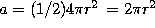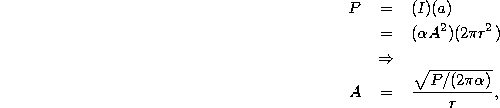Next: Phase Up: Form of solution from Previous: Form of solution from

### Amplitude

If the slit in Figure 1 were truly a point source of waves, then we expect the waves to come out equally in all directions (as indicated in the figure). Moreover, the average total power crossing any half-sphere of radius r (and thus area) must equal the total power P put out by the source. Because the intensity gives the average power per unit time per unit area, we must have, using Eq.2 for the intensity,whereis the proportionality constant from Eq. 2. The basic fact that we have learned the amplitude of the waves from the slit to be inversely proportional to the distance from the slit.

Tomas Arias
Thu Sep 13 15:26:14 EDT 2001